Related Articles
How to set the title and fonts of your Seaborn Chart?
• Last Updated : 25 Feb, 2021

In this article, we are going to see how to set the title and fonts in seaborn chart. Data Visualization is the presentation of data in pictorial format. It is extremely important for Data Analysis, primarily because of the fantastic ecosystem of data-centric Python packages and seaborn is an amazing visualization library for statistical graphics plotting in Python.

Installation:

For python environment :

`pip install seaborn`

For conda environment :

`conda install seaborn`

## Python3

 `# Importing libraries  ``import` `numpy as np  ``import` `seaborn as sns  ``     ` `# Selecting style as white,  ``# dark, whitegrid, darkgrid   ``# or ticks  ``sns.``set``( style ``=` `"white"` `)  ``     ` `# Generate a random univariate   ``# dataset  ``rs ``=` `np.random.RandomState( ``10` `)  ``d ``=` `rs.normal( size ``=` `50` `)  ``     ` `# Plot a simple histogram and kde   ``# with binsize determined automatically  ``sns.distplot(d, kde ``=` `True``, color ``=` `"g"``)`

Output: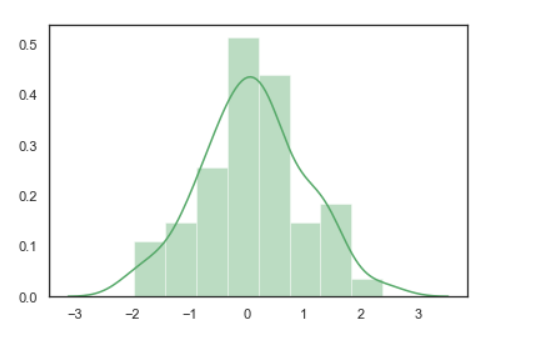Now we can add a title using set_title() function. This function is capable of a set title and font styling.

Syntax: Axes.set_title(label, fontdict)

Parameters:

• label: String
• fontdict: A dictionary controlling the appearance of the title text.

Example 1: Adding title in the seaborn chart.

In this example, we are going to set the title using set_title() function.

Syntax: set_title(“Label”)

Code:

## Python3

 `# Importing libraries  ``import` `numpy as np  ``import` `seaborn as sns  ``     ` `# Selecting style as white,  ``# dark, whitegrid, darkgrid   ``# or ticks  ``sns.``set``( style ``=` `"white"` `)  ``     ` `# Generate a random univariate   ``# dataset  ``rs ``=` `np.random.RandomState( ``10` `)  ``d ``=` `rs.normal( size ``=` `50` `)  ``     ` `# Plot a simple histogram and kde   ``# with binsize determined automatically  ``sns.distplot(d, kde ``=` `True``, color ``=` `"g"``).set_title(``'Seaborn'``)`

Output: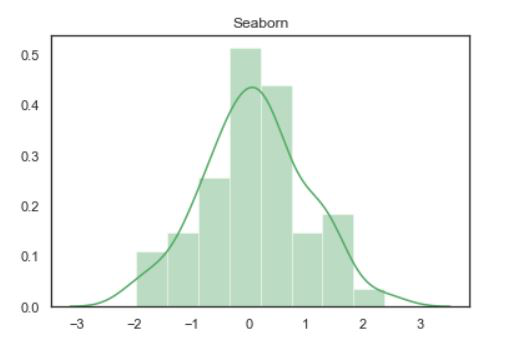Example 2: Increase font size of the title.

Here we are increasing the size of the font using fontsize attributes.

Syntax: set_title(“Label”, fontdict={ ‘fontsize : int’})

Code:

## Python3

 `# Importing libraries  ``import` `numpy as np  ``import` `seaborn as sns  ``     ` `# Selecting style as white,  ``# dark, whitegrid, darkgrid   ``# or ticks  ``sns.``set``( style ``=` `"white"` `)  ``     ` `# Generate a random univariate   ``# dataset  ``rs ``=` `np.random.RandomState( ``10` `)  ``d ``=` `rs.normal( size ``=` `50` `)  ``     ` `# Plot a simple histogram and kde   ``# with binsize determined automatically  ``sns.distplot(d, kde ``=` `True``, color ``=` `"g"``).set_title(``  ``'Seaborn'``, fontdict ``=` `{ ``'fontsize'``: ``30``})`

Output: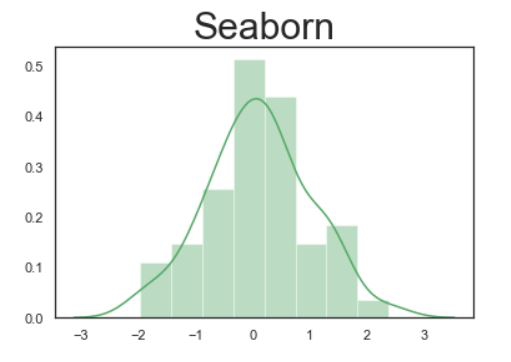Example 3: Setting vertical alignment of a font.

Here we are setting alignment of a font using verticalalignment attributes. Valid verticalalignment value for align; supported values are ‘top’, ‘bottom’, ‘center’, ‘baseline’, ‘center_baseline’.

Syntax: set_title(“Label”, fontdict={ ‘fontsize : int’, verticalalignment =”‘top’, ‘bottom’, ‘center’, ‘baseline’, ‘center_baseline'”})

Code:

## Python3

 `# Importing libraries  ``import` `numpy as np  ``import` `seaborn as sns  ``     ` `# Selecting style as white,  ``# dark, whitegrid, darkgrid   ``# or ticks  ``sns.``set``( style ``=` `"white"` `)  ``     ` `# Generate a random univariate   ``# dataset  ``rs ``=` `np.random.RandomState( ``10` `)  ``d ``=` `rs.normal( size ``=` `50` `)  ``     ` `# Plot a simple histogram and kde   ``# with binsize determined automatically  ``sns.distplot(d, kde ``=` `True``, color ``=` `"g"``).set_title(``  ``'seaborn'``, fontdict``=` `{ ``'fontsize'``: ``30``, ``'verticalalignment'``: ``'bottom'``})`

Output: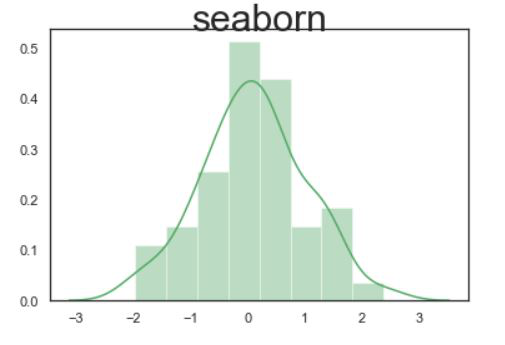Example 4: Setting horizontal alignment.

Here we are setting the alignment of a font using horizontalalignment attributes. Valid horizontalalignment value for align; supported values are center’, ‘right’, ‘left’.

Syntax: set_title(“Label”, fontdict={ ‘fontsize : int’, horizontalalignment =”‘center’, ‘right’, ‘left'”})

Code:

## Python3

 `# Importing libraries  ``import` `numpy as np  ``import` `seaborn as sns      ``     ` `# Selecting style as white,  ``# dark, whitegrid, darkgrid   ``# or ticks  ``sns.``set``( style ``=` `"white"` `)  ``     ` `# Generate a random univariate   ``# dataset  ``rs ``=` `np.random.RandomState( ``10` `)  ``d ``=` `rs.normal( size ``=` `50` `)  ``     ` `# Plot a simple histogram and kde   ``# with binsize determined automatically  ``sns.distplot(d, kde ``=` `True``, color ``=` `"g"``).set_title(``  ``'seaborn'``, fontdict``=` `{ ``'fontsize'``: ``24``, ``'horizontalalignment'``: ``'left'``})`

Output: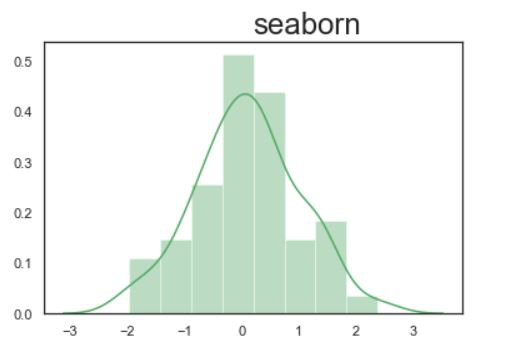Now check for right side alignment using horizontalalignment’: ‘right’:

## Python3

 `# Importing libraries``import` `numpy as np``import` `seaborn as sns`` ` `# Selecting style as white,``# dark, whitegrid, darkgrid``# or ticks``sns.``set``(style``=``"white"``)`` ` `# Generate a random univariate``# dataset``rs ``=` `np.random.RandomState(``10``)``d ``=` `rs.normal(size``=``50``)`` ` `# Plot a simple histogram and kde``# with binsize determined automatically``sns.distplot(d, kde``=``True``, color``=``"g"``).set_title(``  ``'seaborn'``, fontdict``=``{``'fontsize'``: ``24``, ``'horizontalalignment'``: ``'right'``})`

Output: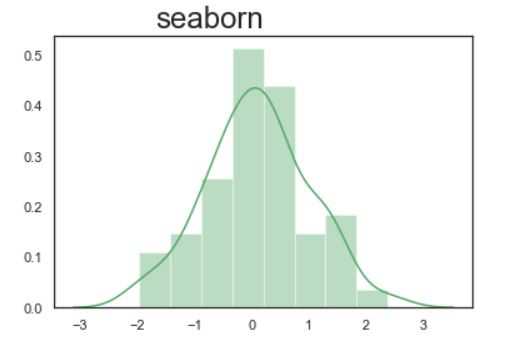Attention geek! Strengthen your foundations with the Python Programming Foundation Course and learn the basics.

To begin with, your interview preparations Enhance your Data Structures concepts with the Python DS Course.

My Personal Notes arrow_drop_up